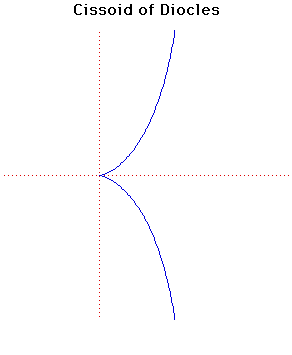# Cissoid of DioclesCartesian equation:
y2 = x3/(2a - x)
Polar equation:
r = 2a tan(θ)sin(θ)

Click below to see one of the Associated curves.

Click THIS LINK to experiment interactively with this curve and its associated curves.

This curve (meaning 'ivy-shaped') was invented by Diocles in about 180 BC in connection with his attempt to duplicate the cube by geometrical methods.

The name first appears in the work of Geminus about 100 years later. Fermat and Roberval constructed the tangent in 1634. Huygens and Wallis found, in 1658, that the area between the curve and its asymptote was 3πa2. From a given point there are either one or three tangents to the cissoid.

The Cissoid of Diocles is the roulette of the vertex of a parabola rolling on an equal parabola.

Newton gave a method of drawing the Cissoid of Diocles using two line segments of equal length at right angles. If they are moved so that one line always passes through a fixed point and the end of the other line segment slides along a straight line then the mid-point of the sliding line segment traces out a Cissoid of Diocles.

Diocles was a contemporary of Nicomedes. He studied the cissoid in his attempt to solve the problem of finding the length of the side of a cube having volume twice that of a given cube. He also studied the problem of Archimedes to cut a sphere by a plane in such a way that the volumes of the segments shall have a given ratio.

It is in commentaries on Archimedes' On the sphere and the cylinder that the cissoid appears and is attributed to Diocles.

The pedal curve of the cissoid, when the pedal point is on the axis beyond the asymptote, at distance from the cusp four times that of the asymptote, is a cardioid.

If the cusp of the cissoid is taken as the centre of inversion, the cissoid inverts to a parabola.

The caustic of the cissoid where the radiant point is taken as (8a, 0) is a cardioid.

If points P and Q are on the cissoid so that PQ subtends a right angle at O then the locus of intersection of the tangents at P and Q lies on the circle with diameter (a/2, 0), (2a, 0).

Other Web site:

JOC/EFR/BS January 1997